Homework Help Question & Answers

# bon will save this response. Question 4 The truss shown below is supported by a roller...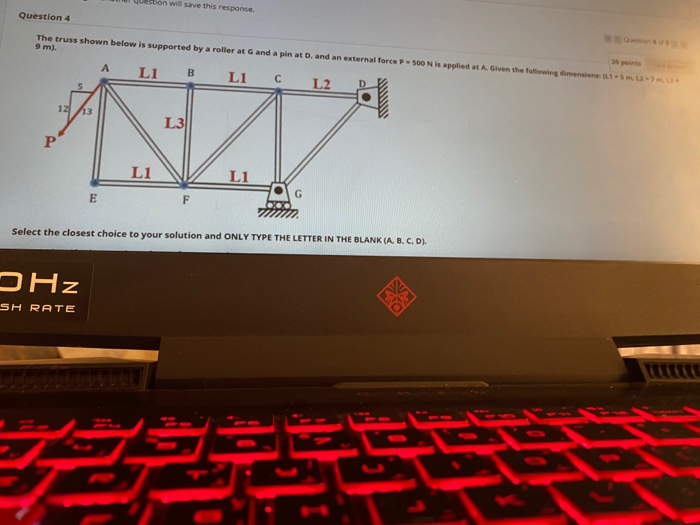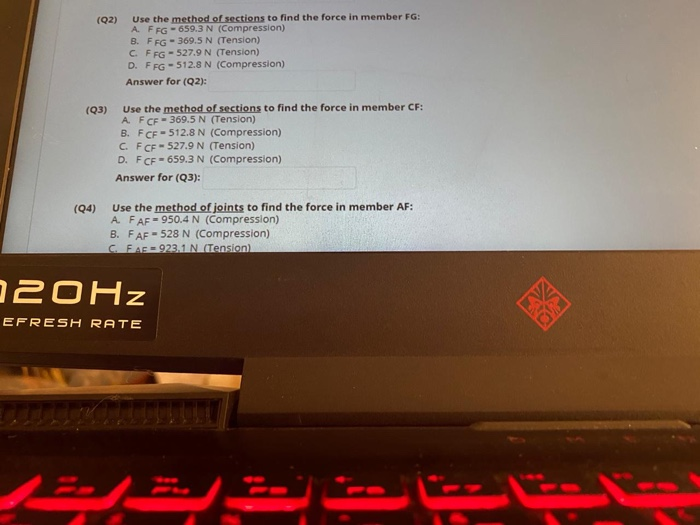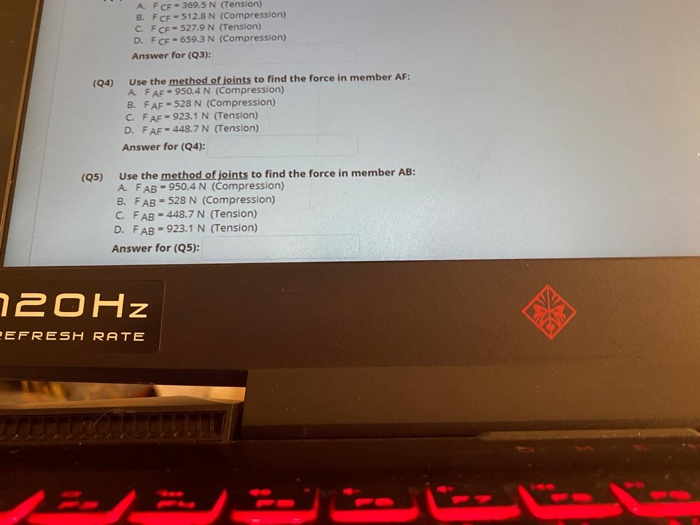bon will save this response. Question 4 The truss shown below is supported by a roller at G and a pin at D, and an external force - 500 N is applied at A. Given the following 9 m). B Lic L2 D L1 12 13 L31 P L1 L1 Select the closest choice to your solution and ONLY TYPE THE LETTER IN THE BLANK (A, B, C, D). DHz SH RATE
(92) Use the method of sections to find the force in member FG: A FFG - 659.3 N (Compression) B. FFG - 369.5 N (Tension) C. FFG - 527.9 N (Tension) D. FFG - 512.8 N (Compression) Answer for (Q2): (Q3) Use the method of sections to find the force in member CF: A FCF - 369.5 N (Tension) B. FCF - 512.8 N (Compression) C. FCF - 527.9 N (Tension) D. FCF - 659.3 N (Compression) Answer for (Q3): (94) Use the method of joints to find the force in member AF: A. FAF = 950.4 N (Compression) B. FAF - 528 N (Compression) C. FAF = 923.1 N (Tension) 20Hz EFRESH RATE
A FCF-369.5 N (Tension) B. FCE - 512.8 N (Compression) C. FCF-527.9 N (Tension) D. FCF - 659.3 N (Compression) Answer for (Q3): (04) Use the method of joints to find the force in member AF: A FAF-950.4 N (Compression) B. FAF - 528 N (Compression) C. FAF-923.1 N (Tension) D. FAF-448.7 N (Tension) Answer for (04): (Q5) Use the method of joints to find the force in member AB: A FAB - 950.4 N (Compression) B. FAB - 528 N (Compression) C. FAB - 448.7 N (Tension) D. FAB - 923.1 N (Tension) Answer for (Q5): 120Hz PEFRESH RATE

#### Homework Answers

Answer #1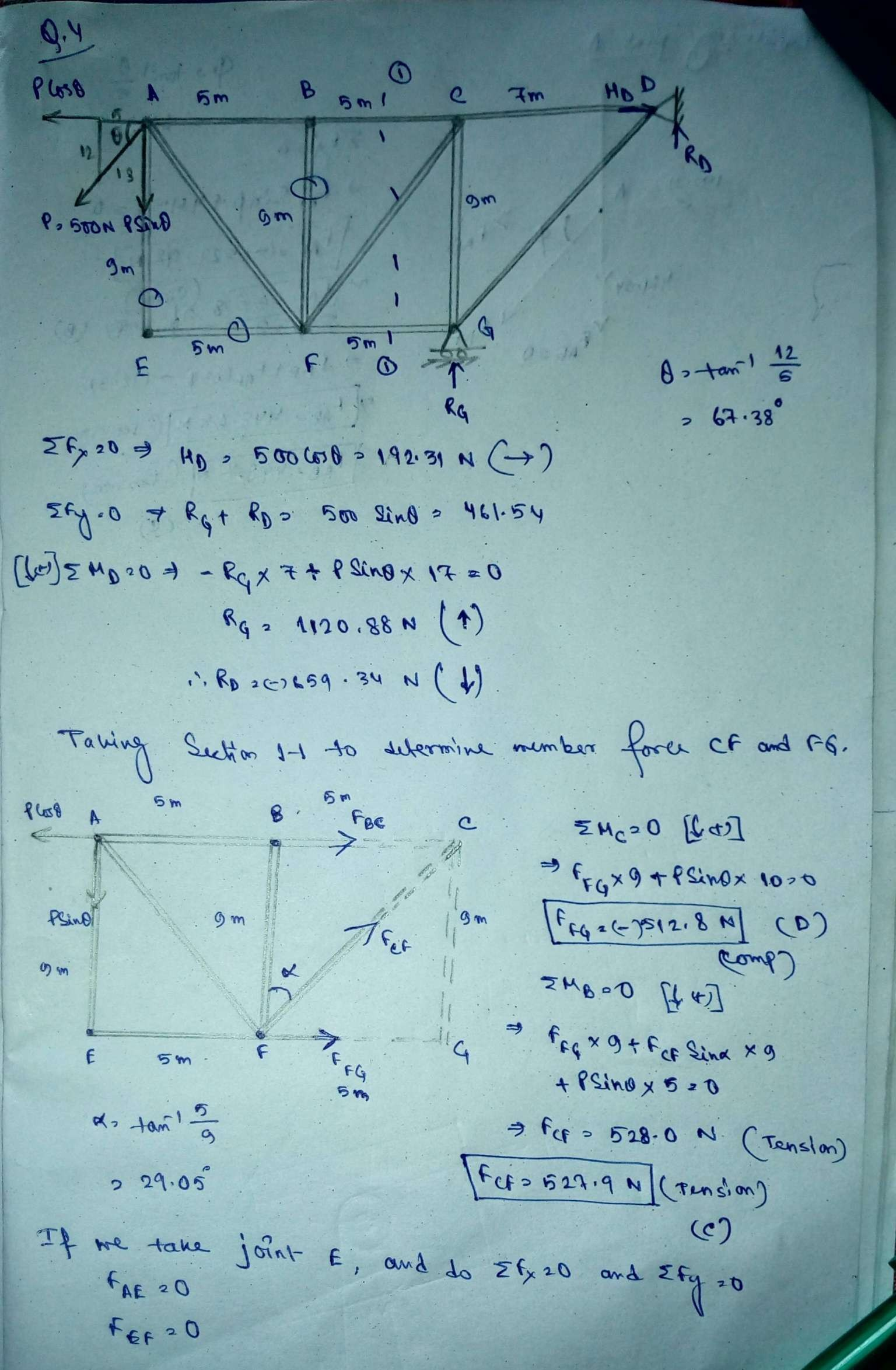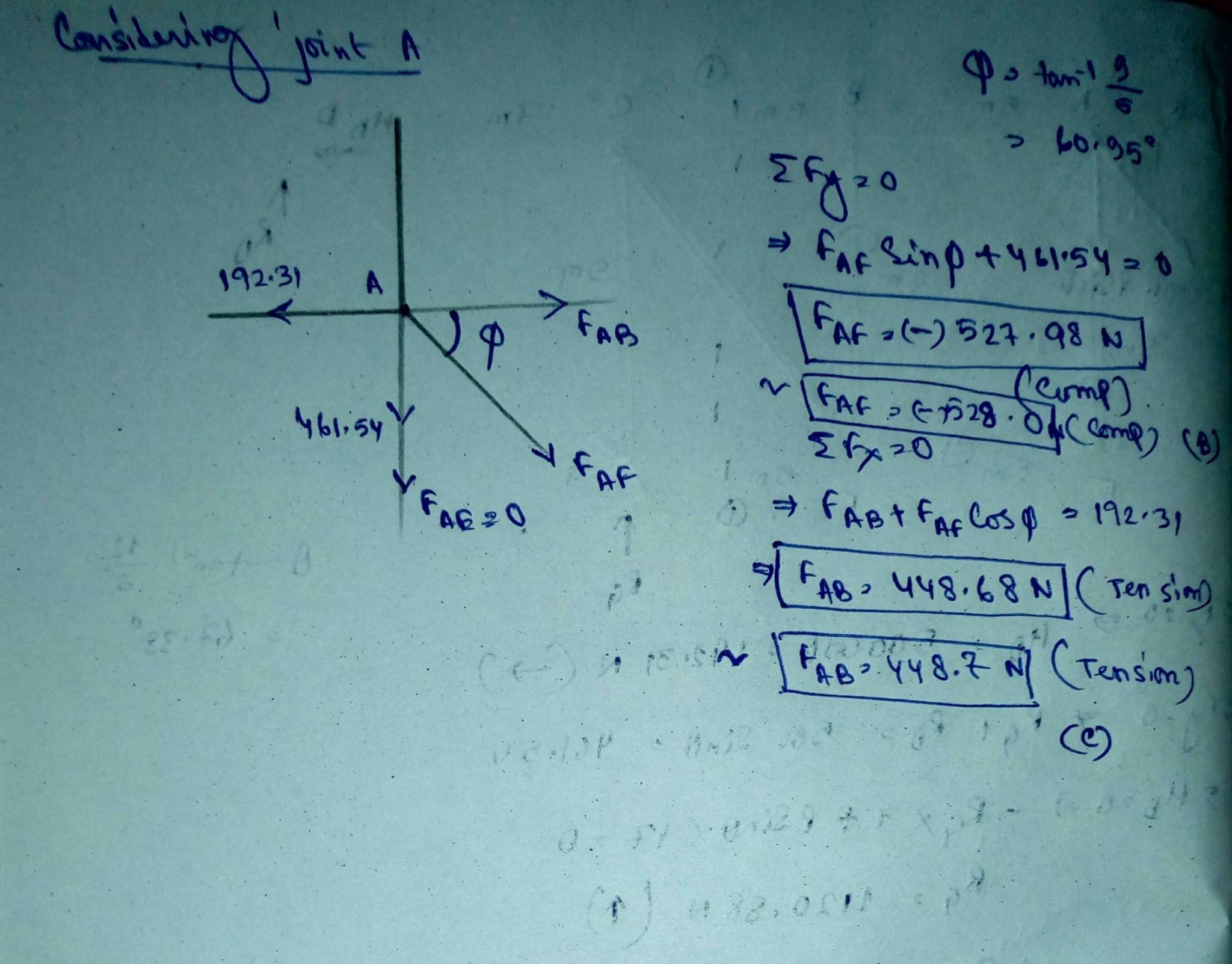Know the answer?
Your Answer:

#### Post as a guest

Your Name:

What's your source?

#### Earn Coin

Coins can be redeemed for fabulous gifts.

Not the answer you're looking for? Ask your own homework help question. Our experts will answer your question WITHIN MINUTES for Free.
Similar Homework Help Questions
• ### Find Fab, Faf, Fbc, Fbf, Fbg, Fcd, Fcg, Fde, Fdg, Fdh, Feh, Ffg, and Fgh HW 11: Method of Jo...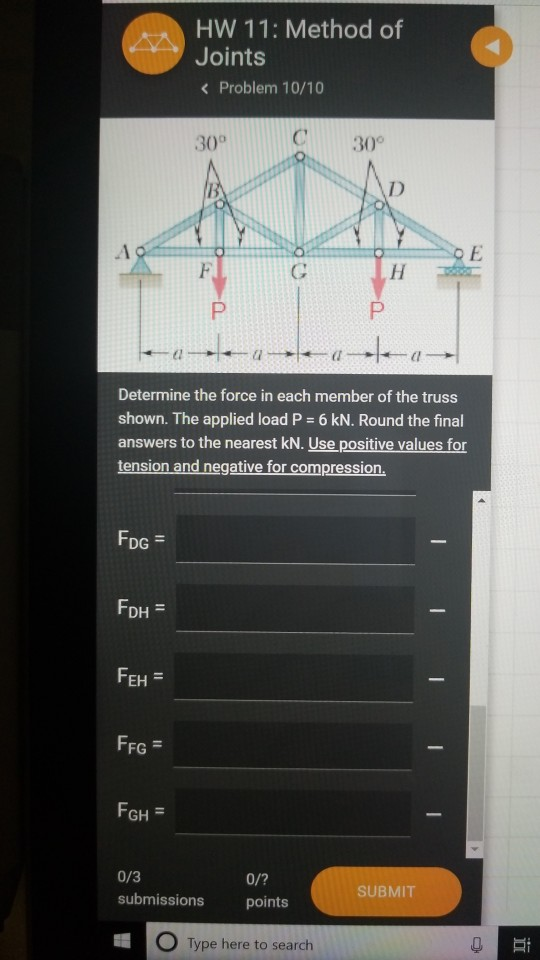Find Fab, Faf, Fbc, Fbf, Fbg, Fcd, Fcg, Fde, Fdg, Fdh, Feh, Ffg, and Fgh HW 11: Method of Joints Problem 10/10 30° C30° Determine the force in each member of the truss shown. The applied load P 6 kN. Round the final answers to the nearest kN. Use positive values for tension and negative for compression. DG = FD EH FFG GH = 0/3 0/? points submissions SUBMIT O Type here to search

• ### A roof truss is loaded and supported as shown in Figure 2. The joints are all...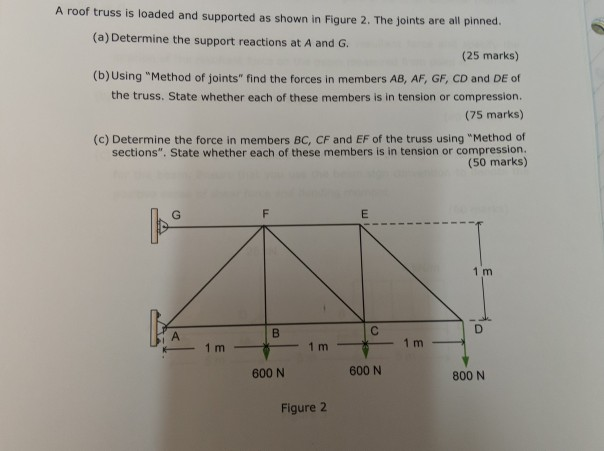A roof truss is loaded and supported as shown in Figure 2. The joints are all pinned. (a) Determine the support reactions at A and G. (25 marks) (b)Using "Method of joints" find the forces in members AB, AF, GF, CD and DE of the truss. State whether each of these members is in tension or compression. (75 marks) (c) Determine the force in members BC, CF and EF of the truss using "Method of sections". State whether each of...

• ### The truss is suspended by two pin joints. Each segment is 3 m wide and 4...

The truss is suspended by two pin joints. Each segment is 3 m wide and 4 m high. The applied force is F = 9 N. What is the force in member 1? Include the sign for tension (positive) or compression (negative). Hint! In some situations it will be best to use the method of sections and in others it will be better to use the method of joints. Answer: 14. [1pt] The force F = 9 N is now...

• ### Will upvote if correct Determine the force in each member of the Howe roof truss shown....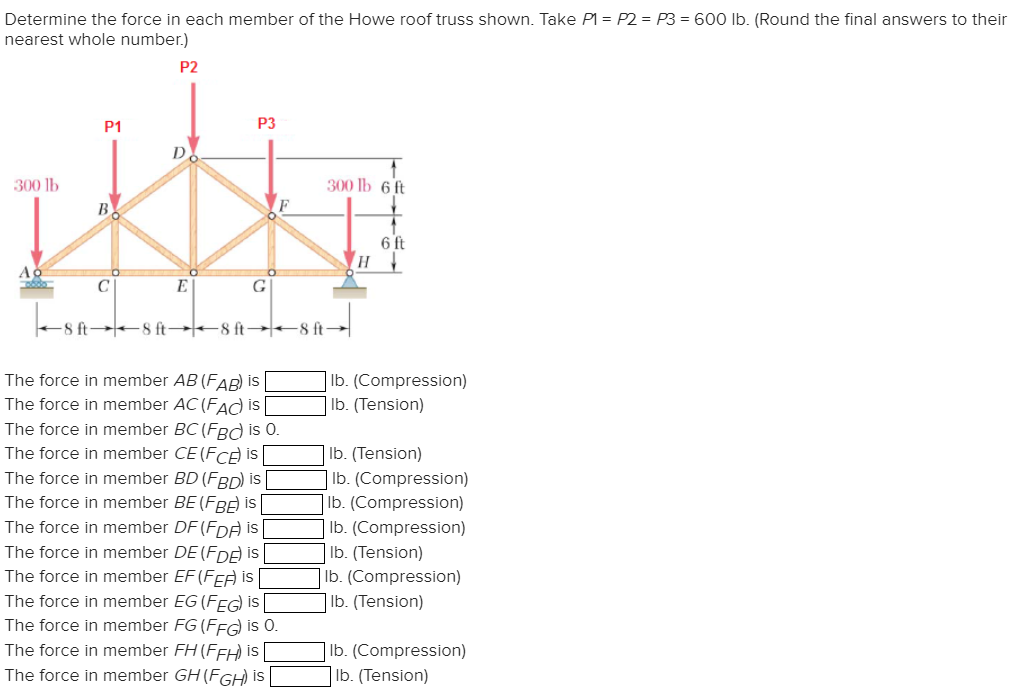Will upvote if correct Determine the force in each member of the Howe roof truss shown. Take P1 = P2 = P3 = 600 lb. (Round the final answers to their nearest whole number.) P2. P1 P3 300 lb 300 lb 6 ft 6 ft E 8f-8f8f8ft l b. (Compression) b. (Tension) The force in member AB (FAB) is The force in member AC(Fad is The force in member BC (Fed is O. The force in member CE(Fce is The...

• ### The truss is suspended by two pin joints. Each segment is 3 m wide and 4...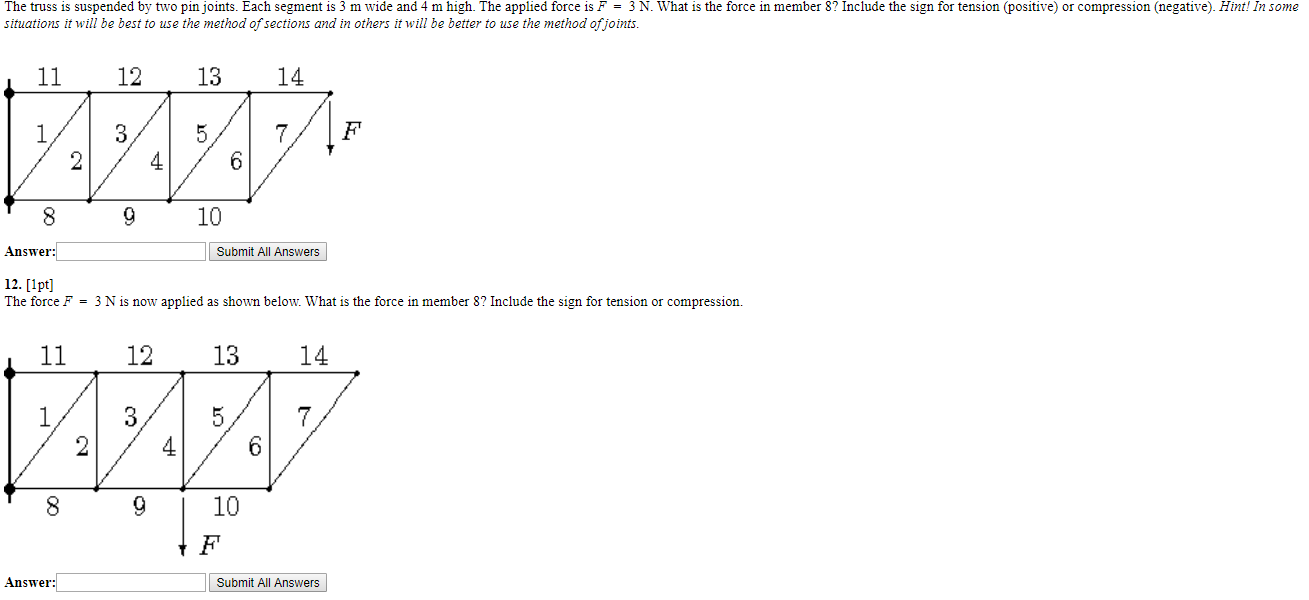The truss is suspended by two pin joints. Each segment is 3 m wide and 4 m high. The applied force is F = 3 N. What is the force in member 8? Include the sign for tension (positive) or compression (negative). Hint! In some situations it will be best to use the method of sections and in others it will be better to use the method of joints. 8 9 10 Answer: Submit All Answers 12. [1pt] The force...

• ### The truss is suspended by two pin joints. Each segment is 3 m wide and 4...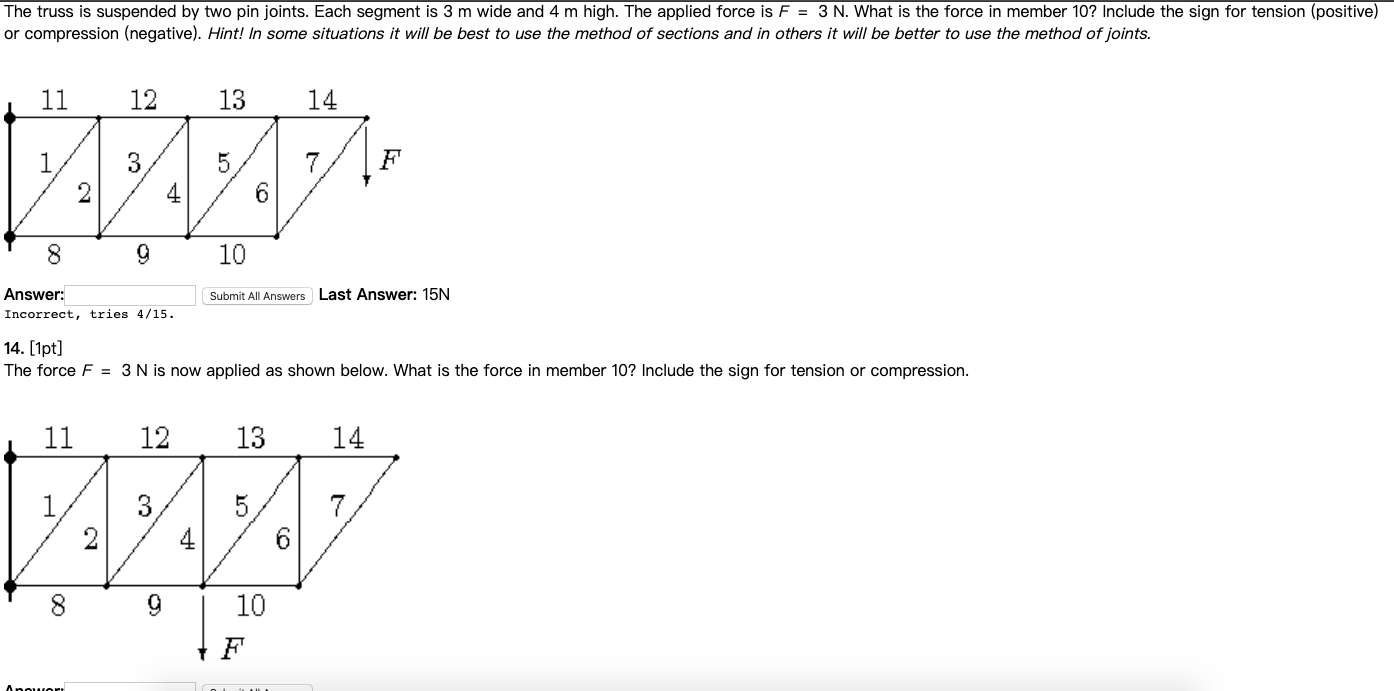The truss is suspended by two pin joints. Each segment is 3 m wide and 4 m high. The applied force is F = 3 N. What is the force in member 10? Include the sign for tension (positive) or compression (negative). Hint! In some situations it will be best to use the method of sections and in others it will be better to use the method of joints. 11 12 13 135 7 24 6 ਨੂੰ ( 8 10...

• ### 13. [1pt] The truss is suspended by two pin joints. Each segment is 3 m wide...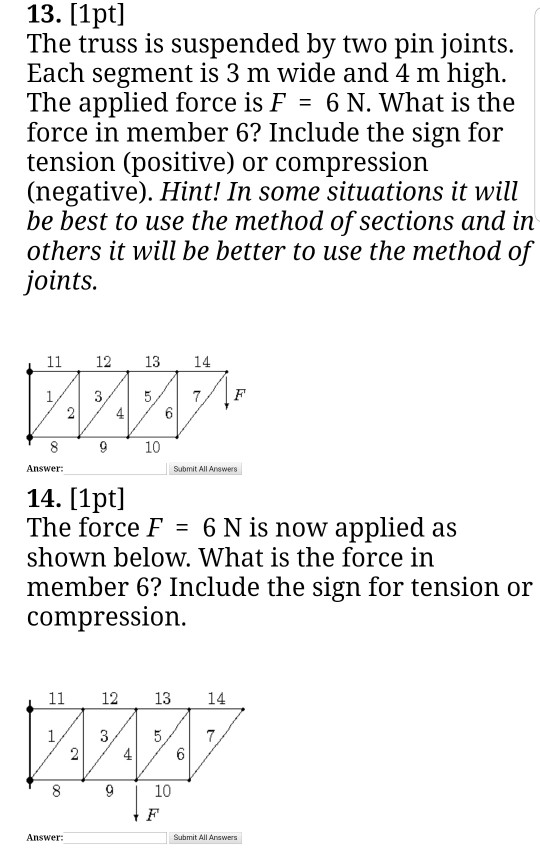13. [1pt] The truss is suspended by two pin joints. Each segment is 3 m wide and 4 m high. The applied force is F = 6 N. What is the force in member 6? Include the sign for tension (positive) or compression (negative). Hint! In some situations it will be best to use the method of sections and in others it will be better to use the method of joints. 11 12 13 2 / 4 9 10 8...

• ### Question Four: For the pin-jointed truss shown in Figure 4, 3m 12 KN 3m 30 kN 3m 15 kN 4m 4m 3m 3m 6m Figure 4. Calcula...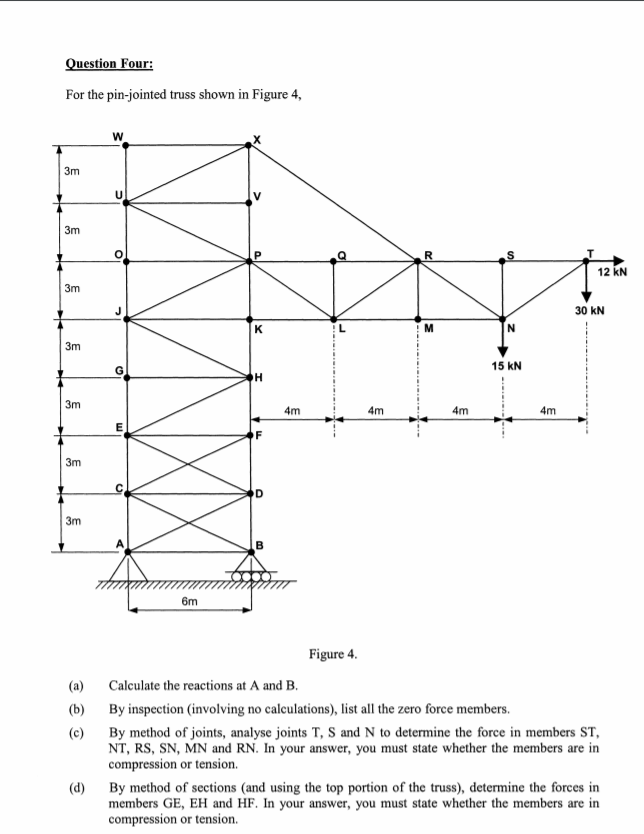Question Four: For the pin-jointed truss shown in Figure 4, 3m 12 KN 3m 30 kN 3m 15 kN 4m 4m 3m 3m 6m Figure 4. Calculate the reactions at A and B. (a) By inspection (involving no calculations), list all the zero force members. (b) (c) By method of joints, analyse joints T, S and N to determine the force in members ST NT, RS, SN, MN and RN. In your answer, you must state whether the members are...

• ### Problem 1: The truss shown is one of several supporting an advertising panel. Using the method...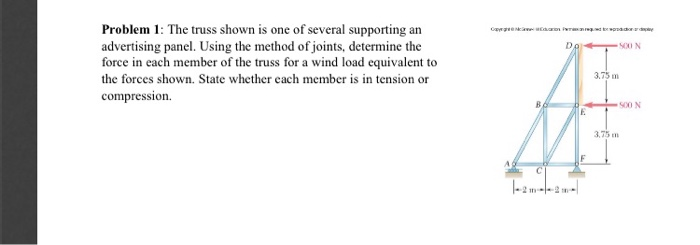Problem 1: The truss shown is one of several supporting an advertising panel. Using the method of joints, determine the force in each member of the truss for a wind load equivalent to Do SO0 N 3,75 m the forces shown. State whether each member is in tension or compression. S00 N 3.75 m C 2 m Problem 1: The truss shown is one of several supporting an advertising panel. Using the method of joints, determine the force in each...

• ### Given: Consider the truss shown below with the loading on joints B and C. Find: Use...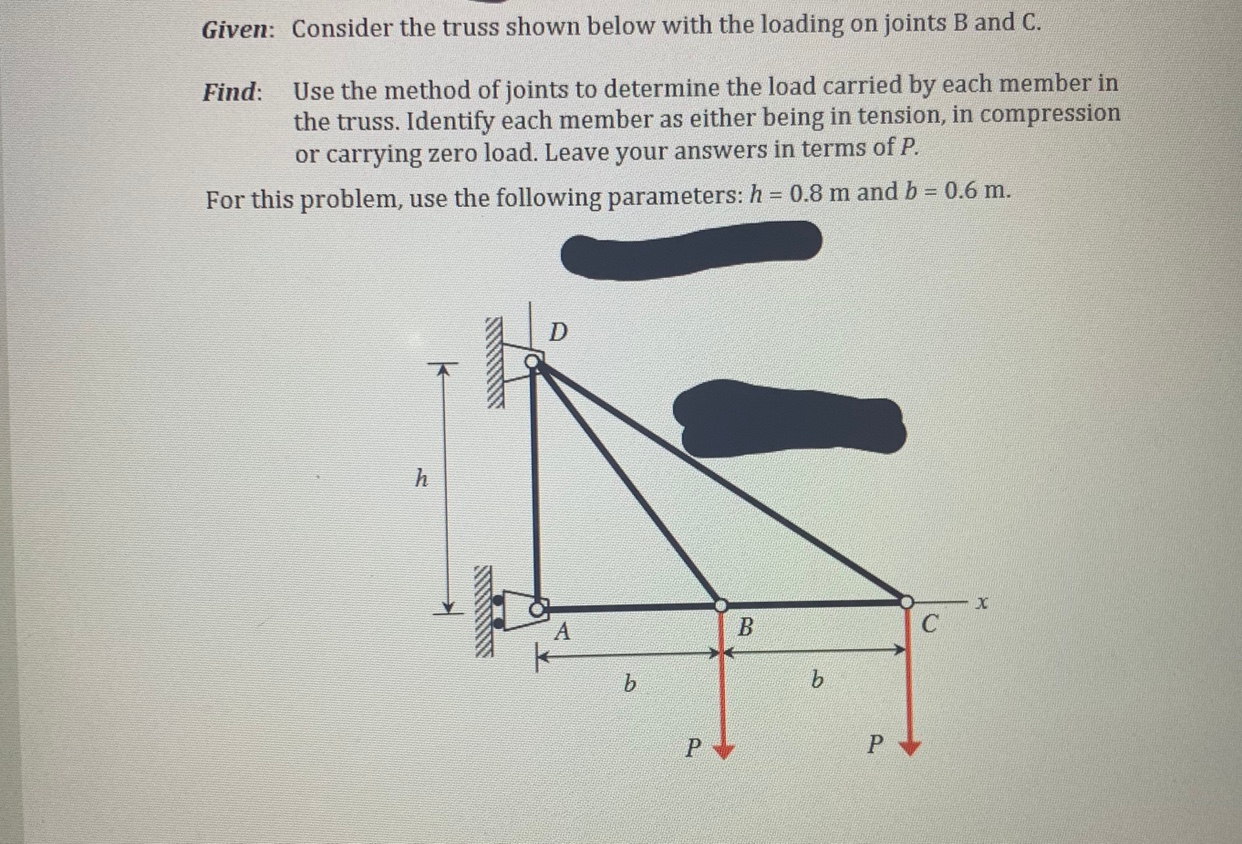Given: Consider the truss shown below with the loading on joints B and C. Find: Use the method of joints to determine the load carried by each member in the truss. Identify each member as either being in tension, in compression or carrying zero load. Leave your answers in terms of P. For this problem, use the following parameters: h = 0.8 m and b = 0.6 m. h X B C b b Р Р

Free Homework App

Scan Your Homework
to Get Instant Free Answers
Need Online Homework Help?

Get Answers For Free
Most questions answered within 3 hours.